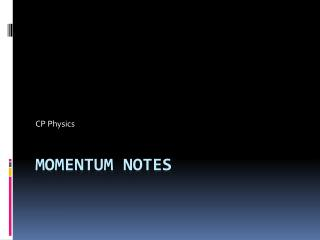# Momentum notes - PowerPoint PPT PresentationDownload PresentationMomentum notes

Momentum notesDownload Presentation## Momentum notes

- - - - - - - - - - - - - - - - - - - - - - - - - - - E N D - - - - - - - - - - - - - - - - - - - - - - - - - - -
##### Presentation Transcript

1. CP Physics Momentum notes

2. Momentum • Idea of inertia in motion • Equation: p = mv, p = momentum, kgm/s • Very massive objects moving slowly can have large momentum while very small objects moving extremely fast can have large momentums • Ex. Aircraft carrier vs. bullet (Mv = mV) • Objects at rest have no momentum

3. Impulse • Impulse = changing momentum – must either change mass or velocity • Usually object’s velocity changes • Changing velocity requires object to be accelerated – requires net force to act on object

4. Impulse, part 2 • Two factors involved in changing momentum • Net force applied - more force = bigger change • Time force is applied – longer time = bigger change • Impulse = force acting over time to change the momentum of an object • Equations: • ∆p = m∆v • ∆p = F∆t • F∆t = m∆v

5. Changing Momentum • Increasing momentum • Apply greatest force possible • Extend time of contact • Largest change in momentum – apply great force over long time • Examples – golfer and baseball players following through when hitting golf ball or baseball

6. Changing Momentum • Decreasing momentum – over long time • Want to lessen force experienced • Extend length of time – will decrease amount of force experienced to less • Examples – car safety devices such as airbags, bumpers, padded dashboards, safety nets

7. Changing Momentum • Decreasing momentum – over short time • If momentum changes over very short time – then force experienced very large • Want very short time if need very large force exerted • Example – breaking blocks in karate